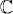# FIRST-ORDER SCALAR EQUATIONS

In this chapter we study the basic properties of first-order scalar ordinary differential equations and their solutions. The first and larger part is devoted to linear equations and various of their basic properties, such as the principle of superposition, Duhamel’s principle, and the concept of stability. In the second part we study briefly nonlinear scalar equations, emphasizing the new behaviors that emerge, and introduce the very useful technique known as the principle of linearization. The scalar equations and their properties are crucial to an understanding of the behavior of more general differential equations.

# 1.1 Constant coefficient linear equations

Consider a complex function y of a real variable t. One of the simplest differential equations that y can obey is given by

(1.1)where λis constant. We want to solve the initial value problem, that is, we want to determine a solution for t ≥ 0 with given initial value

(1.2)Clearly,

is the solution of (1.1), (1.2). Let us discuss the solution under different assumptions for the λ ...

Get Introduction to Numerical Methods for Time Dependent Differential Equations now with the O’Reilly learning platform.

O’Reilly members experience books, live events, courses curated by job role, and more from O’Reilly and nearly 200 top publishers.